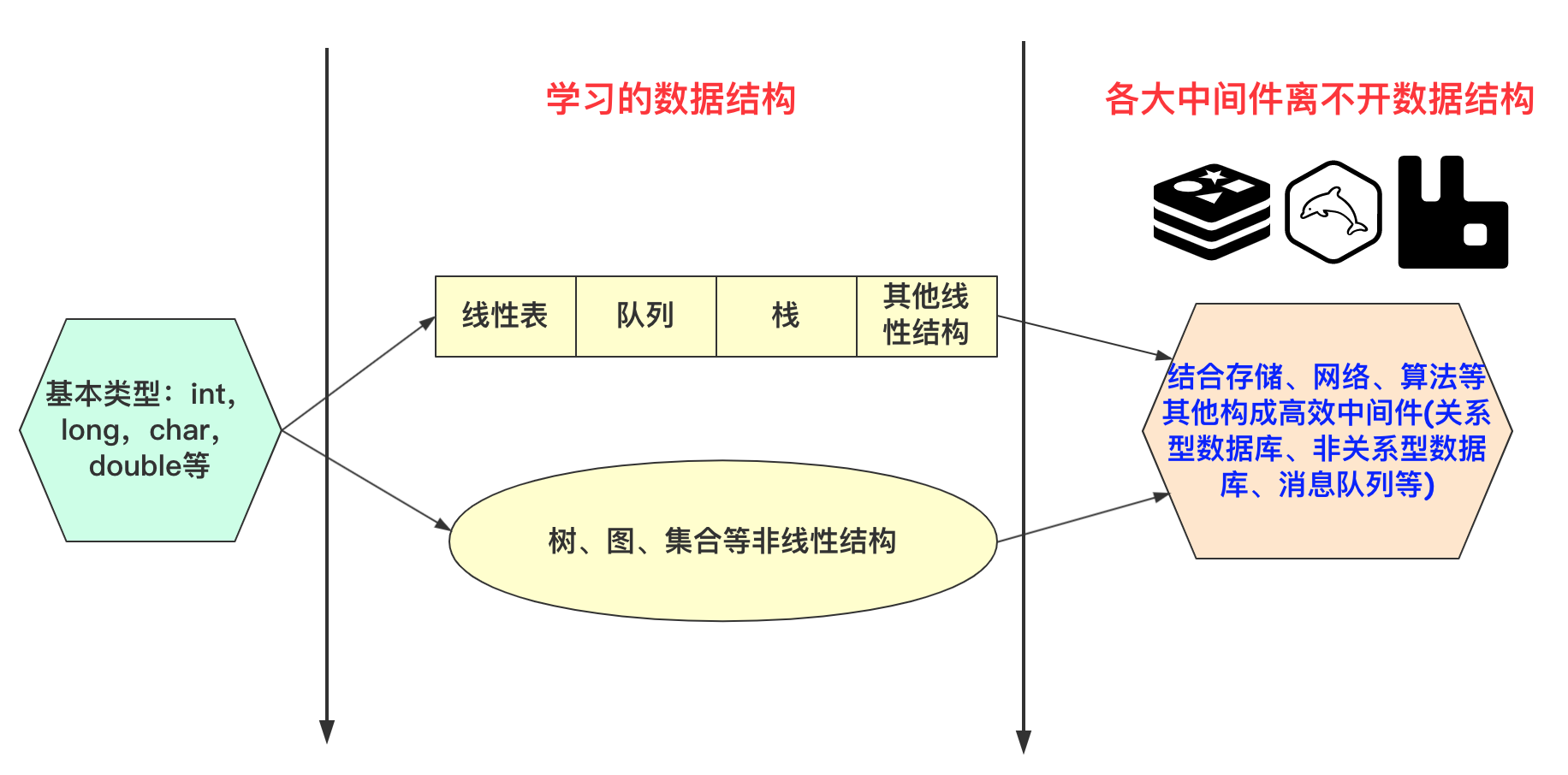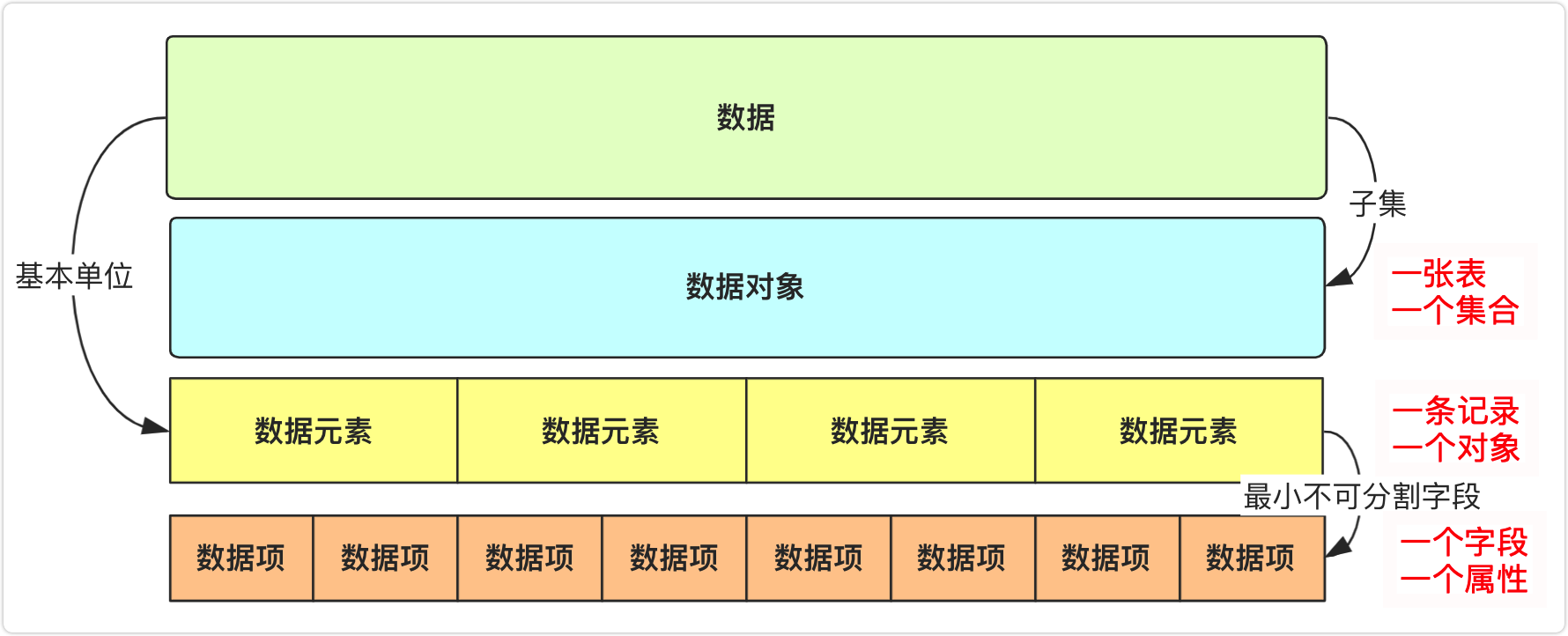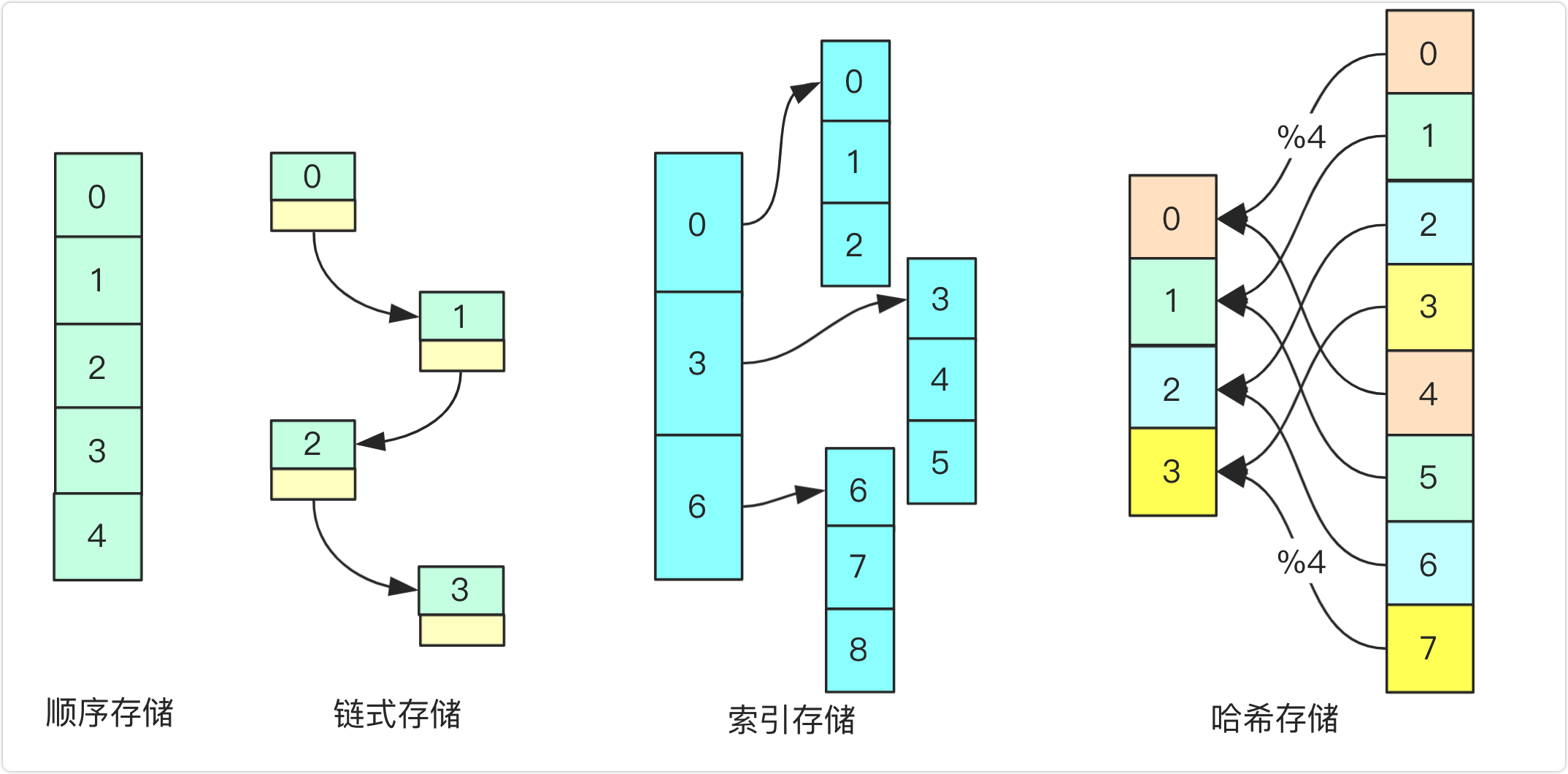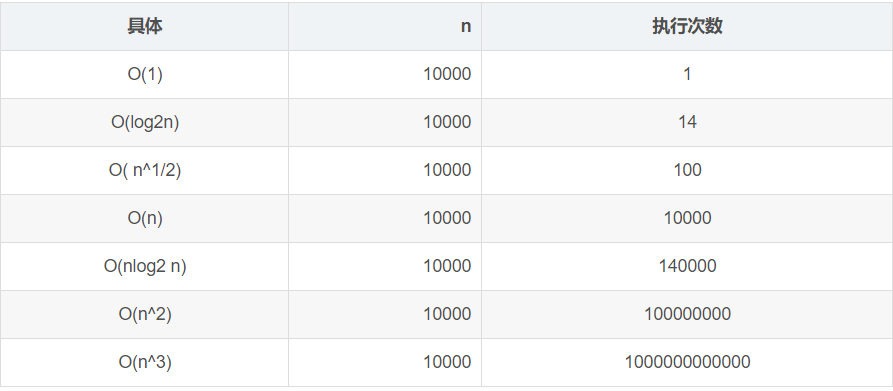# 数据结构与算法必知基础知识bigsai## 数据结构### 相关术语用户信息表users

users 的 pojo 对象

class users{      //略     int id;     String name;     String sex;}//list和woman是数据List<users>list;//数据对象listList<users>woman;//数据对象womanlist.add(new users(001,"bigsai","man"));//添加数据元素 一个users由（001，bigsai，man）三个数据项组成 list.add(new users(002,"smallsai","man"));//数据元素list.add(new users(003,"菜虚鲲","woman"));//数据元素woman.add(list.get(2));//003,"菜虚鲲","woman"三个数据项构成的一个数据元素

原子类型：其值不可再分的类型。比如 int，char，double，float 等。

结构类型：其值可以再分为若干成分的数据类型。比如结构体构造的各种结构等。

### 三要素## 算法分析### 时间复杂度

O(1): 常数函数

• a=15

O(logn): 对数函数

• for(int i=1;i<n;i*=2)分析：假设执行t次使得i=n;有 2^t=n; t=log2~n,为 log 级别时间复杂度为 O(logn)。

• 还有典型的二分查找，拓展欧几里得，快速幂等算法均为 O(logn)。属于高效率算法。

O(n): 线性函数

• for (int i=0;i<n;i++)

• 比较常见，能够良好解决大部分问题。

O(nlogn):

• for (int i=1;i<n;i++) for (int j=1;j<i;j*=2)

• 常见的排序算法很多正常情况都是 nlogn，比如快排、归并排序。这种算法效率大部分也还不错。

O(n^2)

• for(int i=0;i<n;i++)for(int j=0;j<i;j++)

• 其实 O(n^2)的效率就不敢恭维了。对于大的数据 O(n^2)甚至更高次方的执行效果会很差。T(n)=O(m)+O(n)=max(O(m),O(n)); T(n)=O(n)+O(nlogn)=max(O(n),O(nlogn))=O(nlogn);

T(n)=O(m)*O(n)=O(mn)T(n)=O(m)*O(m)=O(m^2)(两层for循环)

## 数据结构与算法学习

### 数据结构

• 单链表(带头结点、不带头结点)设计与实现(增删改查)，双链表设计与实现

• 栈设计与实现(数组和链表)，队列设计与实现(数组和链表)

• 二叉树概念学习，二叉树前序、中序、后序遍历递归、非递归实现 ，层序遍历

• 二叉排序树设计与实现(插入删除)

• 堆(优先队列、堆排序)

• AVL(平衡)树设计与实现(四种自旋方式理解实现)

• 伸展树、红黑树原理概念理解

• B、B+原理概念理解

• 哈夫曼树原理概念理解(贪心策略)

• 哈希(散列表)原理概念理解(几种解决哈希冲突方式)

• 并查集/不相交集合(优化和路径压缩)

• 图论拓扑排序

• 图论 dfs 深度优先遍历、bfs 广度优先遍历

• 最短路径 Dijkstra 算法、Floyd 算法、spfa 算法

• 最小生成树 prim 算法、kruskal 算法

• 其他数据结构线段树、后缀数组等等

### 经典算法

• 递归算法(求阶乘、斐波那契、汉诺塔问题)

• 二分查找

• 分治算法(快排、归并排序、求最近点对等问题)

• 贪心算法(使用较多，区间选点问题，区间覆盖问题)

• 常见动态规划(LCS(最长公共子序列) LIS(最长上升子序列)背包问题等等)

• 回溯算法(经典八皇后问题、全排列问题)

• 位运算常见问题(参考剑指 offer 和 LeetCode 问题)

• 快速幂算法(快速求幂乘、矩阵快速幂)

• kmp 等字符串匹配算法

• 一切其他数论算法(欧几里得、拓展欧几里得、中国剩余定理等等)## 评论